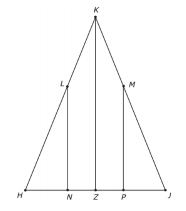D

#### SAT Math

97 viewed last edited 3 years agoSam Peralta
-1In the isosceles triangle, KHJ, HK = 8, NL is perpendicular to HJ and MP is perpendicular to HJ. if K is 10 cm from base HJ and KL = 0.4KH, what is the area of triangle LNH?Mahesh Godavarti
0
Since LN \perp HJ and KZ \perp HJ , we have LN \parallel HJ . Therefore, \triangle LHN \sim \triangle KHZ and \frac{LN}{KZ} = \frac{LH}{KH} = \frac{KH - 0.4KH}{KH} = 0.6 . Therefore, LN = 0.6 KZ and HN = 0.6 HZ . I think HK cannot be equal to 8 as it has to be longer than KZ which is 10cm.Sangeetha Pulapaka
0
Question was wrong HJ = 8 not Hk = 8